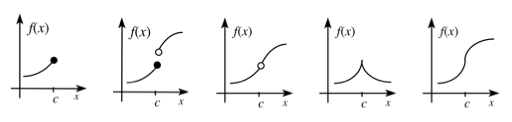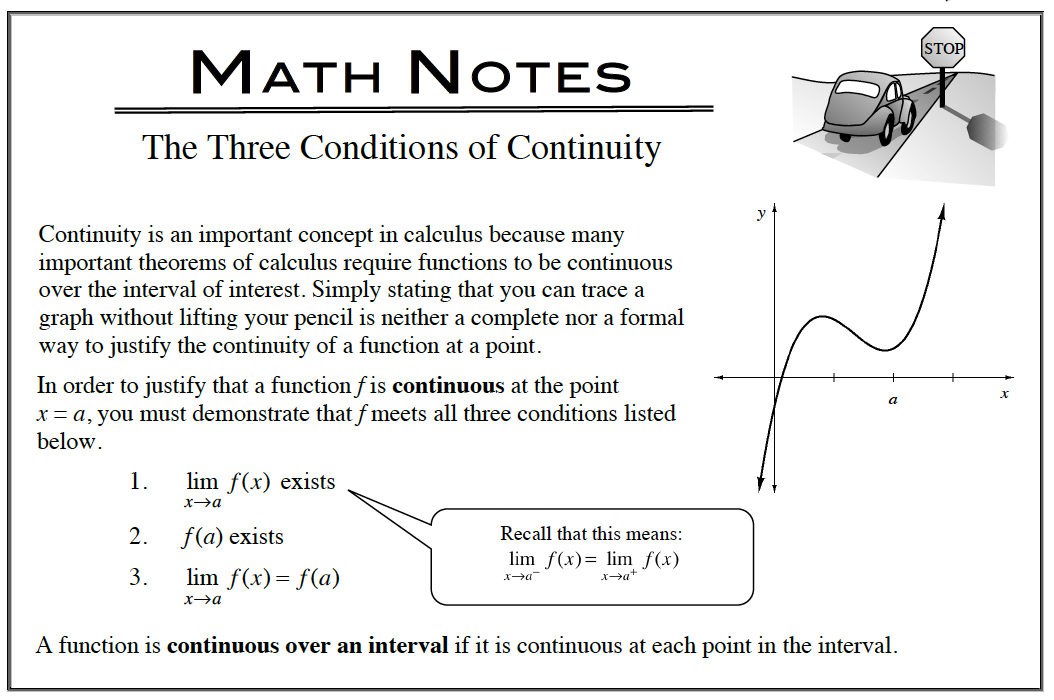### Home > APCALC > Chapter 5 > Lesson 5.4.1 > Problem5-146

5-146.

Multiple Choice: Let f be the function given by f(x) = $|x|$. Which of the following statements about f are true? Homework Help ✎

 I. $f$ is continuous at $x = 0$. II. $f$ is differentiable at $x = 0$. III. $f$ has an absolute minimum at $x = 0$.

Examples of functions that are not differentiable at $x = c$. Notice that some of them are still continuous at $x = c$.A. I only B. II only C. III only D. I and III E. II and III